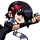General QuestionWoking out if a graph is will be proportional or not?

Asked by weeeee (62) April 8th, 2010

Lets take Ek=(mv^2)/2. What is how to work out kinetic energy Ek, using mass m, speed v.
How would I work out without making a graph if Ek over M is proportional or not?

And I don’t care too much about the answer just so if come across another similar question, lets take Ek=(m^2v)/2 will Ek/m be proportional? I’ll be able to work it out.

I know the basics of a Y=MX+C graph.

Observing members: 0Composing members: 0E is proportional to m if they each have the same exponent. So in the formula for kinetic energy, K = (½)mv^2, K is proportional to m because each has a power of 1. In your second example, E = (½)vm^2, E is not proportional to m; E is proportional to m squared.

Ivan (13439)“Great Answer” (1) Flag as…@Ivan
So it doesn’t matter if there’s a /2 at the end?
so lets make one up Z=xy/x and a graph of z/x and y is constant, would it just be easier to see it as z=x/x .y and because z=x/x the line would decline?

Does it only really matter when something is square when it isn’t constant?

weeeee (62)“Great Answer” (0) Flag as…@weeeee

No, it doesn’t matter. If A is proportional to B, then doubling A will double B; Halving A will halve B, etc. The kinetic energy of a body is proportional to its mass. So if you double the mass, you double the kinetic energy (assuming velocity is unchanged).

Ivan (13439)“Great Answer” (0) Flag as…@Ivan
Last one I’ve been looking in here http://www.khanacademy.org/ for a video to help me out more but can’t seem to find one as I don’t know what it may be called can you help?

weeeee (62)“Great Answer” (0) Flag as…@weeeee

Well, I don’t really know what, specifically, you’re studying. I guess the “Introduction to work and energy” and “conservation of energy” videos might be relevant.

Ivan (13439)“Great Answer” (0) Flag as…or